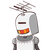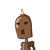# The ratio of two numbers is 5:6. If the larger is 72, what is the smaller?

The ratio of two numbers is 5:6. If the larger is 72, what is the smaller?

### 2 thoughts on “The ratio of two numbers is 5:6. If the larger is 72, what is the smaller?”

1.The ratio of the two numbers is 5:6.

Let the common multiple be ‘x’

The No’s are 5x & 6x

The larger number is 72

Here larger number is 6x

6x = 72

x= 72/6 = 12

x= 12

The smaller number is 5*12 = 60

Ans 60

2.